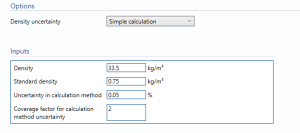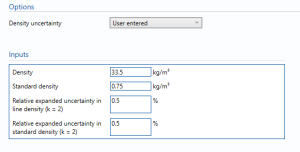# Miscellaneous density

## Options

### Density uncertainty

• User entered
• Simple Calculation

# Miscellaneous density

## Options### Density uncertainty

• Simple Calculation
• User entered

Select the option to get the uncertainty in the density.

## Inputs

Density – Enter the density of gas.

standard density – enter the density of gas at standard conditions.

uncertainty in calculation method – uncertainty in the calculation used.

Coverage factor for calculation method uncertainty – the coverage factor to accompany the uncertainty in calculation method.

Note: only applicable if simple calculation is selected from the drop down box.

Relative expanded uncertainty in line density – the percentage uncertainty in the density of gas flowing through the gas turbine with 95% confidence level.

Note: only applicable if User entered is selected from the drop down box.

Relative expanded uncertainty in standard density – the percentage uncertainty in the density of gas at standard conditions with 95% confidence level.

Note: only applicable if User entered is selected from the drop down box.

## Uncertainty Budget

The uncertainty budget table shows a break down of the different components that contribute to the overall calculated uncertainty.The values input into the uncertainty budget are derived from the measured pressure and the transmitter specific values relating to its calibration and specification. These values are taken in as the expanded uncertainties and are divided by a coverage factor to gain the standard uncertainty.

The coverage factor is determined by the probability distribution that best suits that uncertainty component. The standard uncertainty is then multiplied by the sensitivity value then squared. This is done for each component that contributes to the overall uncertainty in density or standard density. The Standard Uncertainty in density or standard density is the square root of the sum of each component variance as shown in the following equation:### Calculated UncertaintyThe Expanded Uncertainty is the Standard Uncertainty multiplied by the coverage factor (k). The coverage factor is defaulted to k = 2 (equivalent to a confidence level of approximately 95%).

The Relative Uncertainty is the Expanded Uncertainty divided by the density or standard density depending on the parameter.

Back to Uncertainty Modules